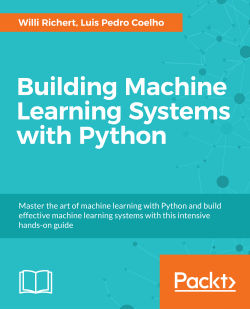# Building Machine Learning Systems with Python4.8 (11 reviews total)
By Willi Richert , Luis Pedro Coelho
What do you get with a Packt Subscription?

• Constantly updated with 100+ new titles each month
• Breadth and depth in over 1,000+ technologies

Machine learning, the field of building systems that learn from data, is exploding on the Web and elsewhere. Python is a wonderful language in which to develop machine learning applications. As a dynamic language, it allows for fast exploration and experimentation and an increasing number of machine learning libraries are developed for Python.

Building Machine Learning system with Python shows you exactly how to find patterns through raw data. The book starts by brushing up on your Python ML knowledge and introducing libraries, and then moves on to more serious projects on datasets, Modelling, Recommendations, improving recommendations through examples and sailing through sound and image processing in detail.

Using open-source tools and libraries, readers will learn how to apply methods to text, images, and sounds. You will also learn how to evaluate, compare, and choose machine learning techniques.

Written for Python programmers, Building Machine Learning Systems with Python teaches you how to use open-source libraries to solve real problems with machine learning. The book is based on real-world examples that the user can build on.

Readers will learn how to write programs that classify the quality of StackOverflow answers or whether a music file is Jazz or Metal. They will learn regression, which is demonstrated on how to recommend movies to users. Advanced topics such as topic modeling (finding a text’s most important topics), basket analysis, and cloud computing are covered as well as many other interesting aspects.

Building Machine Learning Systems with Python will give you the tools and understanding required to build your own systems, which are tailored to solve your problems.

Publication date:
July 2013
Publisher
Packt
Pages
290
ISBN
9781782161400

## Chapter 1. Getting Started with Python Machine Learning

Machine learning (ML) teaches machines how to carry out tasks by themselves. It is that simple. The complexity comes with the details, and that is most likely the reason you are reading this book.

Maybe you have too much data and too little insight, and you hoped that using machine learning algorithms will help you solve this challenge. So you started to dig into random algorithms. But after some time you were puzzled: which of the myriad of algorithms should you actually choose?

Or maybe you are broadly interested in machine learning and have been reading a few blogs and articles about it for some time. Everything seemed to be magic and cool, so you started your exploration and fed some toy data into a decision tree or a support vector machine. But after you successfully applied it to some other data, you wondered, was the whole setting right? Did you get the optimal results? And how do you know there are no better algorithms? Or whether your data was "the right one"?

Welcome to the club! We, the authors, were at those stages once upon a time, looking for information that tells the real story behind the theoretical textbooks on machine learning. It turned out that much of that information was "black art", not usually taught in standard textbooks. So, in a sense, we wrote this book to our younger selves; a book that not only gives a quick introduction to machine learning, but also teaches you lessons that we have learned along the way. We hope that it will also give you, the reader, a smoother entry into one of the most exciting fields in Computer Science.

## Machine learning and Python – the dream team

The goal of machine learning is to teach machines (software) to carry out tasks by providing them with a couple of examples (how to do or not do a task). Let us assume that each morning when you turn on your computer, you perform the same task of moving e-mails around so that only those e-mails belonging to a particular topic end up in the same folder. After some time, you feel bored and think of automating this chore. One way would be to start analyzing your brain and writing down all the rules your brain processes while you are shuffling your e-mails. However, this will be quite cumbersome and always imperfect. While you will miss some rules, you will over-specify others. A better and more future-proof way would be to automate this process by choosing a set of e-mail meta information and body/folder name pairs and let an algorithm come up with the best rule set. The pairs would be your training data, and the resulting rule set (also called model) could then be applied to future e-mails that we have not yet seen. This is machine learning in its simplest form.

Of course, machine learning (often also referred to as data mining or predictive analysis) is not a brand new field in itself. Quite the contrary, its success over recent years can be attributed to the pragmatic way of using rock-solid techniques and insights from other successful fields; for example, statistics. There, the purpose is for us humans to get insights into the data by learning more about the underlying patterns and relationships. As you read more and more about successful applications of machine learning (you have checked out kaggle.com already, haven't you?), you will see that applied statistics is a common field among machine learning experts.

As you will see later, the process of coming up with a decent ML approach is never a waterfall-like process. Instead, you will see yourself going back and forth in your analysis, trying out different versions of your input data on diverse sets of ML algorithms. It is this explorative nature that lends itself perfectly to Python. Being an interpreted high-level programming language, it may seem that Python was designed specifically for the process of trying out different things. What is more, it does this very fast. Sure enough, it is slower than C or similar statically-typed programming languages; nevertheless, with a myriad of easy-to-use libraries that are often written in C, you don't have to sacrifice speed for agility.

## What the book will teach you (and what it will not)

This book will give you a broad overview of the types of learning algorithms that are currently used in the diverse fields of machine learning and what to watch out for when applying them. From our own experience, however, we know that doing the "cool" stuff—using and tweaking machine learning algorithms such as support vector machines (SVM), nearest neighbor search (NNS), or ensembles thereof—will only consume a tiny fraction of the overall time of a good machine learning expert. Looking at the following typical workflow, we see that most of our time will be spent in rather mundane tasks:

1. Reading the data and cleaning it.

2. Exploring and understanding the input data.

3. Analyzing how best to present the data to the learning algorithm.

4. Choosing the right model and learning algorithm.

5. Measuring the performance correctly.

When talking about exploring and understanding the input data, we will need a bit of statistics and basic math. But while doing this, you will see that those topics, which seemed so dry in your math class, can actually be really exciting when you use them to look at interesting data.

The journey begins when you read in the data. When you have to face issues such as invalid or missing values, you will see that this is more an art than a precise science. And a very rewarding one, as doing this part right will open your data to more machine learning algorithms, and thus increase the likelihood of success.

With the data being ready in your program's data structures, you will want to get a real feeling of what kind of animal you are working with. Do you have enough data to answer your questions? If not, you might want to think about additional ways to get more of it. Do you maybe even have too much data? Then you probably want to think about how best to extract a sample of it.

Often you will not feed the data directly into your machine learning algorithm. Instead, you will find that you can refine parts of the data before training. Many times, the machine learning algorithm will reward you with increased performance. You will even find that a simple algorithm with refined data generally outperforms a very sophisticated algorithm with raw data. This part of the machine learning workflow is called feature engineering, and it is generally a very exciting and rewarding challenge. Creative and intelligent that you are, you will immediately see the results.

Choosing the right learning algorithm is not simply a shootout of the three or four that are in your toolbox (there will be more algorithms in your toolbox that you will see). It is more of a thoughtful process of weighing different performance and functional requirements. Do you need fast results and are willing to sacrifice quality? Or would you rather spend more time to get the best possible result? Do you have a clear idea of the future data or should you be a bit more conservative on that side?

Finally, measuring the performance is the part where most mistakes are waiting for the aspiring ML learner. There are easy ones, such as testing your approach with the same data on which you have trained. But there are more difficult ones; for example, when you have imbalanced training data. Again, data is the part that determines whether your undertaking will fail or succeed.

We see that only the fourth point is dealing with the fancy algorithms. Nevertheless, we hope that this book will convince you that the other four tasks are not simply chores, but can be equally important if not more exciting. Our hope is that by the end of the book you will have truly fallen in love with data instead of learned algorithms.

In the rest of this chapter, we will set up and get to know the basic Python libraries, NumPy and SciPy, and then train our first machine learning using scikit-learn. During this endeavor, we will introduce basic ML concepts that will later be used throughout the book. The rest of the chapters will then go into more detail through the five steps described earlier, highlighting different aspects of machine learning in Python using diverse application scenarios.

## What to do when you are stuck

We try to convey every idea necessary to reproduce the steps throughout this book. Nevertheless, there will be situations when you might get stuck. The reasons might range from simple typos over odd combinations of package versions to problems in understanding.

In such a situation, there are many different ways to get help. Most likely, your problem will already have been raised and solved in the following excellent Q&A sites:

• http://metaoptimize.com/qa – This Q&A site is laser-focused on machine learning topics. For almost every question, it contains above-average answers from machine learning experts. Even if you don't have any questions, it is a good habit to check it out every now and then and read through some of the questions and answers.

• http://stats.stackexchange.com – This Q&A site, named Cross Validated, is similar to MetaOptimized, but focuses more on statistics problems.

• http://stackoverflow.com – This Q&A site is similar to the previous ones, but with a broader focus on general programming topics. It contains, for example, more questions on some of the packages that we will use in this book (SciPy and Matplotlib).

• `#machinelearning` on Freenode – This IRC channel is focused on machine learning topics. It is a small but very active and helpful community of machine learning experts.

• http://www.TwoToReal.com – This is an instant Q&A site written by us, the authors, to support you in topics that don't fit in any of the above buckets. If you post your question, we will get an instant message; if any of us are online, we will be drawn into a chat with you.

As stated at the beginning, this book tries to help you get started quickly on your machine learning journey. We therefore highly encourage you to build up your own list of machine learning-related blogs and check them out regularly. This is the best way to get to know what works and what does not.

The only blog we want to highlight right here is http://blog.kaggle.com, the blog of the Kaggle company, which is carrying out machine learning competitions (more links are provided in Appendix A, Where to Learn More about Machine Learning). Typically, they encourage the winners of the competitions to write down how they approached the competition, what strategies did not work, and how they arrived at the winning strategy. If you don't read anything else, fine; but this is a must.

## Getting started

Assuming that you have already installed Python (everything at least as recent as 2.7 should be fine), we need to install NumPy and SciPy for numerical operations as well as Matplotlib for visualization.

### Introduction to NumPy, SciPy, and Matplotlib

Before we can talk about concrete machine learning algorithms, we have to talk about how best to store the data we will chew through. This is important as the most advanced learning algorithm will not be of any help to us if they will never finish. This may be simply because accessing the data is too slow. Or maybe its representation forces the operating system to swap all day. Add to this that Python is an interpreted language (a highly optimized one, though) that is slow for many numerically heavy algorithms compared to C or Fortran. So we might ask why on earth so many scientists and companies are betting their fortune on Python even in the highly computation-intensive areas?

The answer is that in Python, it is very easy to offload number-crunching tasks to the lower layer in the form of a C or Fortran extension. That is exactly what NumPy and SciPy do (http://scipy.org/install.html). In this tandem, NumPy provides the support of highly optimized multidimensional arrays, which are the basic data structure of most state-of-the-art algorithms. SciPy uses those arrays to provide a set of fast numerical recipes. Finally, Matplotlib (http://matplotlib.org/) is probably the most convenient and feature-rich library to plot high-quality graphs using Python.

### Installing Python

Luckily, for all the major operating systems, namely Windows, Mac, and Linux, there are targeted installers for NumPy, SciPy, and Matplotlib. If you are unsure about the installation process, you might want to install Enthought Python Distribution (https://www.enthought.com/products/epd_free.php) or Python(x,y) (http://code.google.com/p/pythonxy/wiki/Downloads), which come with all the earlier mentioned packages included.

### Chewing data efficiently with NumPy and intelligently with SciPy

Let us quickly walk through some basic NumPy examples and then take a look at what SciPy provides on top of it. On the way, we will get our feet wet with plotting using the marvelous Matplotlib package.

You will find more interesting examples of what NumPy can offer at http://www.scipy.org/Tentative_NumPy_Tutorial.

You will also find the book NumPy Beginner's Guide - Second Edition, Ivan Idris, Packt Publishing very valuable. Additional tutorial style guides are at http://scipy-lectures.github.com; you may also visit the official SciPy tutorial at http://docs.scipy.org/doc/scipy/reference/tutorial.

In this book, we will use NumPy Version 1.6.2 and SciPy Version 0.11.0.

### Learning NumPy

So let us import NumPy and play a bit with it. For that, we need to start the Python interactive shell.

```>>> import numpy
>>> numpy.version.full_version
1.6.2```

As we do not want to pollute our namespace, we certainly should not do the following:

```>>> from numpy import *
```

The `numpy.array` array will potentially shadow the array package that is included in standard Python. Instead, we will use the following convenient shortcut:

```>>> import numpy as np
>>> a = np.array([0,1,2,3,4,5])
>>> a
array([0, 1, 2, 3, 4, 5])
>>> a.ndim
1
>>> a.shape
(6,)```

We just created an array in a similar way to how we would create a list in Python. However, NumPy arrays have additional information about the shape. In this case, it is a one-dimensional array of five elements. No surprises so far.

We can now transform this array in to a 2D matrix.

```>>> b = a.reshape((3,2))
>>> b
array([[0, 1],
[2, 3],
[4, 5]])
>>> b.ndim
2
>>> b.shape
(3, 2)```

The funny thing starts when we realize just how much the NumPy package is optimized. For example, it avoids copies wherever possible.

```>>> b=77
>>> b
array([[ 0,  1],
[77,  3],
[ 4,  5]])
>>> a
array([ 0,  1, 77,  3,  4,  5])```

In this case, we have modified the value `2` to `77` in `b`, and we can immediately see the same change reflected in `a` as well. Keep that in mind whenever you need a true copy.

```>>> c = a.reshape((3,2)).copy()
>>> c
array([[ 0,  1],
[77,  3],
[ 4,  5]])
>>> c = -99
>>> a
array([ 0,  1, 77,  3,  4,  5])
>>> c
array([[-99,   1],
[ 77,   3],
[  4,   5]])```

Here, `c` and `a` are totally independent copies.

Another big advantage of NumPy arrays is that the operations are propagated to the individual elements.

```>>> a*2
array([ 2,  4,  6,  8, 10])
>>> a**2
array([ 1,  4,  9, 16, 25])
Contrast that to ordinary Python lists:
>>> [1,2,3,4,5]*2
[1, 2, 3, 4, 5, 1, 2, 3, 4, 5]
>>> [1,2,3,4,5]**2
Traceback (most recent call last):
File "<stdin>", line 1, in <module>
TypeError: unsupported operand type(s) for ** or pow(): 'list' and 'int'```

Of course, by using NumPy arrays we sacrifice the agility Python lists offer. Simple operations like adding or removing are a bit complex for NumPy arrays. Luckily, we have both at our disposal, and we will use the right one for the task at hand.

#### Indexing

Part of the power of NumPy comes from the versatile ways in which its arrays can be accessed.

In addition to normal list indexing, it allows us to use arrays themselves as indices.

```>>> a[np.array([2,3,4])]
array([77,  3,  4])```

In addition to the fact that conditions are now propagated to the individual elements, we gain a very convenient way to access our data.

```>>> a>4
array([False, False,  True, False, False,  True], dtype=bool)
>>> a[a>4]
array([77,  5])```

This can also be used to trim outliers.

```>>> a[a>4] = 4
>>> a
array([0, 1, 4, 3, 4, 4])```

As this is a frequent use case, there is a special clip function for it, clipping the values at both ends of an interval with one function call as follows:

```>>> a.clip(0,4)
array([0, 1, 4, 3, 4, 4])```

#### Handling non-existing values

The power of NumPy's indexing capabilities comes in handy when preprocessing data that we have just read in from a text file. It will most likely contain invalid values, which we will mark as not being a real number using `numpy.NAN` as follows:

```c = np.array([1, 2, np.NAN, 3, 4]) # let's pretend we have read this from a text file
>>> c
array([  1.,   2.,  nan,   3.,   4.])
>>> np.isnan(c)
array([False, False,  True, False, False], dtype=bool)
>>> c[~np.isnan(c)]
array([ 1.,  2.,  3.,  4.])
>>> np.mean(c[~np.isnan(c)])
2.5```

#### Comparing runtime behaviors

Let us compare the runtime behavior of NumPy with normal Python lists. In the following code, we will calculate the sum of all squared numbers of 1 to 1000 and see how much time the calculation will take. We do it 10000 times and report the total time so that our measurement is accurate enough.

```import timeit
normal_py_sec = timeit.timeit('sum(x*x for x in xrange(1000))',
number=10000)
naive_np_sec = timeit.timeit('sum(na*na)',
setup="import numpy as np; na=np.arange(1000)",
number=10000)
good_np_sec = timeit.timeit('na.dot(na)',
setup="import numpy as np; na=np.arange(1000)",
number=10000)

print("Normal Python: %f sec"%normal_py_sec)
print("Naive NumPy: %f sec"%naive_np_sec)
print("Good NumPy: %f sec"%good_np_sec)

Normal Python: 1.157467 sec
Naive NumPy: 4.061293 sec
Good NumPy: 0.033419 sec```

We make two interesting observations. First, just using NumPy as data storage (Naive NumPy) takes 3.5 times longer, which is surprising since we believe it must be much faster as it is written as a C extension. One reason for this is that the access of individual elements from Python itself is rather costly. Only when we are able to apply algorithms inside the optimized extension code do we get speed improvements, and tremendous ones at that: using the `dot()` function of NumPy, we are more than 25 times faster. In summary, in every algorithm we are about to implement, we should always look at how we can move loops over individual elements from Python to some of the highly optimized NumPy or SciPy extension functions.

However, the speed comes at a price. Using NumPy arrays, we no longer have the incredible flexibility of Python lists, which can hold basically anything. NumPy arrays always have only one datatype.

```>>> a = np.array([1,2,3])
>>> a.dtype
dtype('int64')```

If we try to use elements of different types, NumPy will do its best to coerce them to the most reasonable common datatype:

```>>> np.array([1, "stringy"])
array(['1', 'stringy'], dtype='|S8')
>>> np.array([1, "stringy", set([1,2,3])])
array([1, stringy, set([1, 2, 3])], dtype=object)```

### Learning SciPy

On top of the efficient data structures of NumPy, SciPy offers a magnitude of algorithms working on those arrays. Whatever numerical-heavy algorithm you take from current books on numerical recipes, you will most likely find support for them in SciPy in one way or another. Whether it is matrix manipulation, linear algebra, optimization, clustering, spatial operations, or even Fast Fourier transformation, the toolbox is readily filled. Therefore, it is a good habit to always inspect the `scipy` module before you start implementing a numerical algorithm.

For convenience, the complete namespace of NumPy is also accessible via SciPy. So, from now on, we will use NumPy's machinery via the SciPy namespace. You can check this easily by comparing the function references of any base function; for example:

```>>> import scipy, numpy
>>> scipy.version.full_version
0.11.0
>>> scipy.dot is numpy.dot
True```

The diverse algorithms are grouped into the following toolboxes:

SciPy package

Functionality

`cluster`

Hierarchical clustering (`cluster.hierarchy`)

Vector quantization / K-Means (`cluster.vq`)

`constants`

Physical and mathematical constants

Conversion methods

`fftpack`

Discrete Fourier transform algorithms

`integrate`

Integration routines

`interpolate`

Interpolation (linear, cubic, and so on)

`io`

Data input and output

`linalg`

Linear algebra routines using the optimized BLAS and LAPACK libraries

`maxentropy`

Functions for fitting maximum entropy models

`ndimage`

n-dimensional image package

`odr`

Orthogonal distance regression

`optimize`

Optimization (finding minima and roots)

`signal`

Signal processing

`sparse`

Sparse matrices

`spatial`

Spatial data structures and algorithms

`special`

Special mathematical functions such as Bessel or Jacobian

`stats`

Statistics toolkit

The toolboxes most interesting to our endeavor are `scipy.stats`, `scipy.interpolate`, `scipy.cluster`, and `scipy.signal`. For the sake of brevity, we will briefly explore some features of the `stats` package and leave the others to be explained when they show up in the chapters.

## Our first (tiny) machine learning application

Let us get our hands dirty and have a look at our hypothetical web startup, MLAAS, which sells the service of providing machine learning algorithms via HTTP. With the increasing success of our company, the demand for better infrastructure also increases to serve all incoming web requests successfully. We don't want to allocate too many resources as that would be too costly. On the other hand, we will lose money if we have not reserved enough resources for serving all incoming requests. The question now is, when will we hit the limit of our current infrastructure, which we estimated being 100,000 requests per hour. We would like to know in advance when we have to request additional servers in the cloud to serve all the incoming requests successfully without paying for unused ones.

We have collected the web stats for the last month and aggregated them in `ch01/data/web_traffic.tsv` (`tsv` because it contains tab separated values). They are stored as the number of hits per hour. Each line contains consecutive hours and the number of web hits in that hour.

The first few lines look like the following: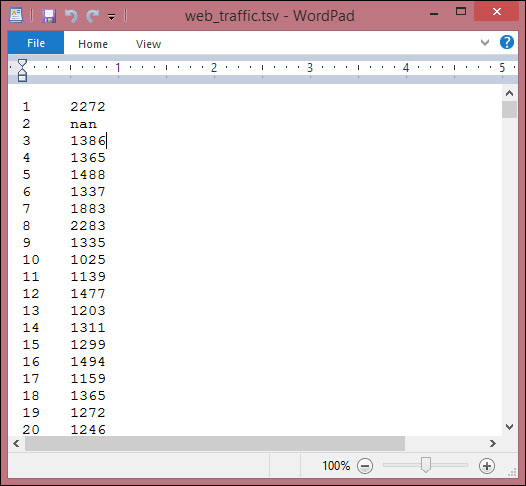Using SciPy's `genfromtxt()`, we can easily read in the data.

```import scipy as sp
data = sp.genfromtxt("web_traffic.tsv", delimiter="\t")```

We have to specify tab as the delimiter so that the columns are correctly determined.

A quick check shows that we have correctly read in the data.

```>>> print(data[:10])
[[  1.00000000e+00   2.27200000e+03]
[  2.00000000e+00              nan]
[  3.00000000e+00   1.38600000e+03]
[  4.00000000e+00   1.36500000e+03]
[  5.00000000e+00   1.48800000e+03]
[  6.00000000e+00   1.33700000e+03]
[  7.00000000e+00   1.88300000e+03]
[  8.00000000e+00   2.28300000e+03]
[  9.00000000e+00   1.33500000e+03]
[  1.00000000e+01   1.02500000e+03]]
>>> print(data.shape)
(743, 2)```

We have 743 data points with two dimensions.

### Preprocessing and cleaning the data

It is more convenient for SciPy to separate the dimensions into two vectors, each of size 743. The first vector, `x`, will contain the hours and the other, `y`, will contain the web hits in that particular hour. This splitting is done using the special index notation of SciPy, using which we can choose the columns individually.

```x = data[:,0]
y = data[:,1]```

### Note

There is much more to the way data can be selected from a SciPy array. Check out http://www.scipy.org/Tentative_NumPy_Tutorial for more details on indexing, slicing, and iterating.

One caveat is that we still have some values in `y` that contain invalid values, `nan`. The question is, what can we do with them? Let us check how many hours contain invalid data.

```>>> sp.sum(sp.isnan(y))
8```

We are missing only 8 out of 743 entries, so we can afford to remove them. Remember that we can index a SciPy array with another array. `sp.isnan(y)` returns an array of Booleans indicating whether an entry is not a number. Using `~`, we logically negate that array so that we choose only those elements from `x` and `y` where `y` does contain valid numbers.

```x = x[~sp.isnan(y)]
y = y[~sp.isnan(y)]```

To get a first impression of our data, let us plot the data in a scatter plot using Matplotlib. Matplotlib contains the `pyplot` package, which tries to mimic Matlab's interface—a very convenient and easy-to-use one (you will find more tutorials on plotting at http://matplotlib.org/users/pyplot_tutorial.html).

```import matplotlib.pyplot as plt
plt.scatter(x,y)
plt.title("Web traffic over the last month")
plt.xlabel("Time")
plt.ylabel("Hits/hour")
plt.xticks([w*7*24 for w in range(10)],
['week %i'%w for w in range(10)])
plt.autoscale(tight=True)
plt.grid()
plt.show()```

In the resulting chart, we can see that while in the first weeks the traffic stayed more or less the same, the last week shows a steep increase: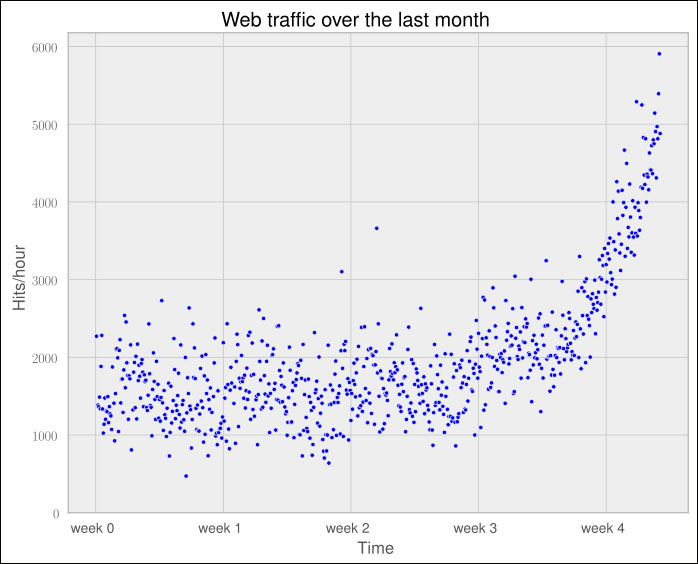### Choosing the right model and learning algorithm

Now that we have a first impression of the data, we return to the initial question: how long will our server handle the incoming web traffic? To answer this we have to:

• Find the real model behind the noisy data points

• Use the model to extrapolate into the future to find the point in time where our infrastructure has to be extended

#### Before building our first model

When we talk about models, you can think of them as simplified theoretical approximations of the complex reality. As such there is always some inferiority involved, also called the approximation error. This error will guide us in choosing the right model among the myriad of choices we have. This error will be calculated as the squared distance of the model's prediction to the real data. That is, for a learned model function, `f`, the error is calculated as follows:

```def error(f, x, y):
return sp.sum((f(x)-y)**2)```

The vectors `x` and `y` contain the web stats data that we have extracted before. It is the beauty of SciPy's vectorized functions that we exploit here with `f(x)`. The trained model is assumed to take a vector and return the results again as a vector of the same size so that we can use it to calculate the difference to `y`.

#### Starting with a simple straight line

Let us assume for a second that the underlying model is a straight line. The challenge then is how to best put that line into the chart so that it results in the smallest approximation error. SciPy's `polyfit()` function does exactly that. Given data `x` and `y` and the desired order of the polynomial (straight line has order `1`), it finds the model function that minimizes the error function defined earlier.

`fp1, residuals, rank, sv, rcond = sp.polyfit(x, y, 1, full=True)`

The `polyfit()` function returns the parameters of the fitted model function, `fp1`; and by setting `full` to `True`, we also get additional background information on the fitting process. Of it, only residuals are of interest, which is exactly the error of the approximation.

```>>> print("Model parameters: %s" %  fp1)
Model parameters: [   2.59619213  989.02487106]
>>> print(res)
[  3.17389767e+08]```

This means that the best straight line fit is the following function:

`f(x) = 2.59619213 * x + 989.02487106.`

We then use `poly1d()` to create a model function from the model parameters.

```>>> f1 = sp.poly1d(fp1)
>>> print(error(f1, x, y))
317389767.34```

We have used `full=True` to retrieve more details on the fitting process. Normally, we would not need it, in which case only the model parameters would be returned.

### Note

In fact, what we do here is simple curve fitting. You can find out more about it on Wikipedia by going to http://en.wikipedia.org/wiki/Curve_fitting.

We can now use `f1()` to plot our first trained model. In addition to the earlier plotting instructions, we simply add the following:

```fx = sp.linspace(0,x[-1], 1000) # generate X-values for plotting
plt.plot(fx, f1(fx), linewidth=4)
plt.legend(["d=%i" % f1.order], loc="upper left")```

The following graph shows our first trained model: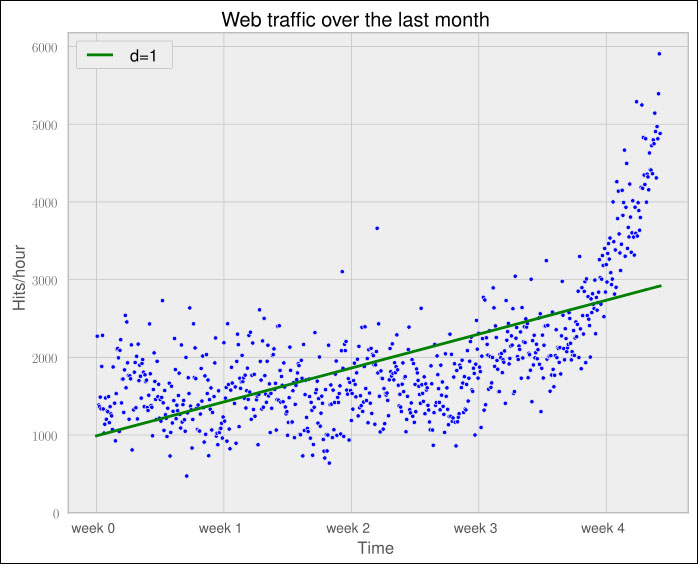It seems like the first four weeks are not that far off, although we clearly see that there is something wrong with our initial assumption that the underlying model is a straight line. Plus, how good or bad actually is the error of 317,389,767.34?

The absolute value of the error is seldom of use in isolation. However, when comparing two competing models, we can use their errors to judge which one of them is better. Although our first model clearly is not the one we would use, it serves a very important purpose in the workflow: we will use it as our baseline until we find a better one. Whatever model we will come up with in the future, we will compare it against the current baseline.

Let us now fit a more complex model, a polynomial of degree 2, to see whether it better "understands" our data:

```>>> f2p = sp.polyfit(x, y, 2)
>>> print(f2p)
array([  1.05322215e-02,  -5.26545650e+00,   1.97476082e+03])
>>> f2 = sp.poly1d(f2p)
>>> print(error(f2, x, y))
179983507.878```

The following chart shows the model we trained before (straight line of one degree) with our newly trained, more complex model with two degrees (dashed):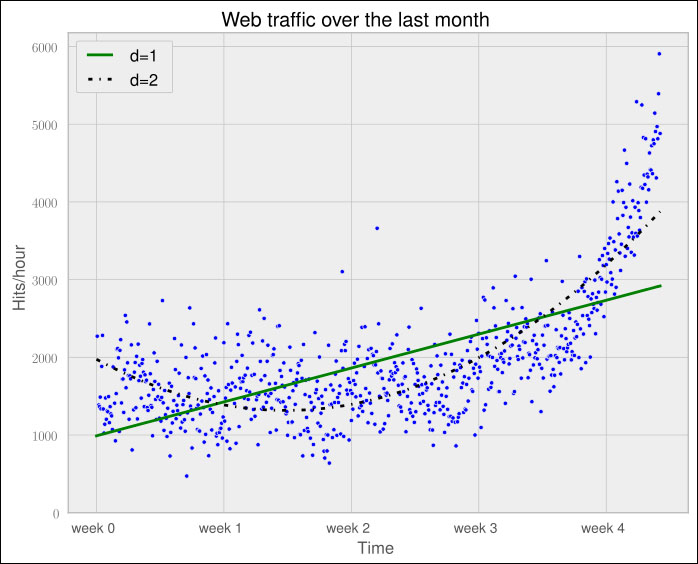The error is 179,983,507.878, which is almost half the error of the straight-line model. This is good; however, it comes with a price. We now have a more complex function, meaning that we have one more parameter to tune inside `polyfit()`. The fitted polynomial is as follows:

`f(x) = 0.0105322215 * x**2  - 5.26545650 * x + 1974.76082`

So, if more complexity gives better results, why not increase the complexity even more? Let's try it for degree 3, 10, and 100.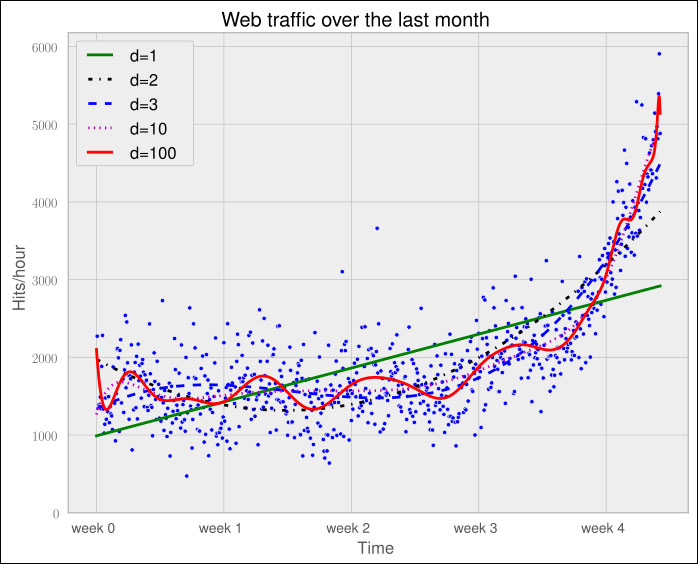The more complex the data gets, the curves capture it and make it fit better. The errors seem to tell the same story.

```Error d=1: 317,389,767.339778
Error d=2: 179,983,507.878179
Error d=3: 139,350,144.031725
Error d=10: 121,942,326.363461
Error d=100: 109,318,004.475556```

However, taking a closer look at the fitted curves, we start to wonder whether they also capture the true process that generated this data. Framed differently, do our models correctly represent the underlying mass behavior of customers visiting our website? Looking at the polynomial of degree 10 and 100, we see wildly oscillating behavior. It seems that the models are fitted too much to the data. So much that it is now capturing not only the underlying process but also the noise. This is called overfitting.

At this point, we have the following choices:

• Selecting one of the fitted polynomial models.

• Switching to another more complex model class; splines?

• Thinking differently about the data and starting again.

Of the five fitted models, the first-order model clearly is too simple, and the models of order 10 and 100 are clearly overfitting. Only the second- and third-order models seem to somehow match the data. However, if we extrapolate them at both borders, we see them going berserk.

Switching to a more complex class also seems to be the wrong way to go about it. What arguments would back which class? At this point, we realize that we probably have not completely understood our data.

#### Stepping back to go forward – another look at our data

So, we step back and take another look at the data. It seems that there is an inflection point between weeks 3 and 4. So let us separate the data and train two lines using week 3.5 as a separation point. We train the first line with the data up to week 3, and the second line with the remaining data.

```inflection = 3.5*7*24 # calculate the inflection point in hours
xa = x[:inflection] # data before the inflection point
ya = y[:inflection]
xb = x[inflection:] # data after
yb = y[inflection:]

fa = sp.poly1d(sp.polyfit(xa, ya, 1))
fb = sp.poly1d(sp.polyfit(xb, yb, 1))

fa_error = error(fa, xa, ya)
fb_error = error(fb, xb, yb)
print("Error inflection=%f" % (fa + fb_error))
Error inflection=156,639,407.701523```

Plotting the two models for the two data ranges gives the following chart: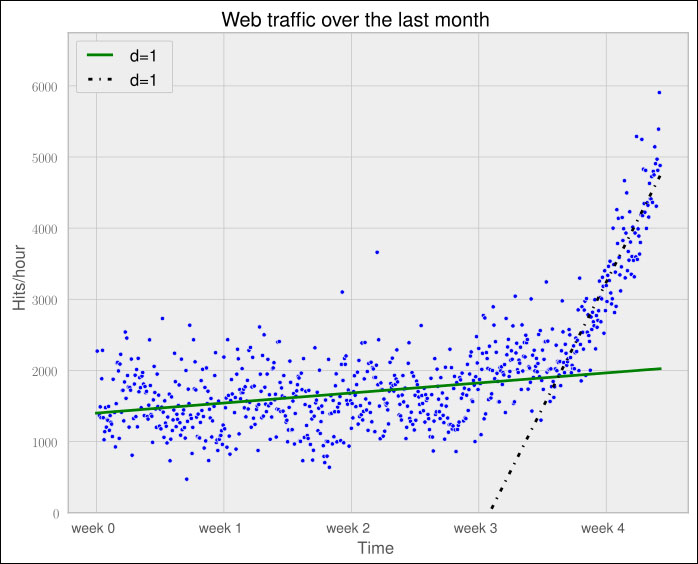Clearly, the combination of these two lines seems to be a much better fit to the data than anything we have modeled before. But still, the combined error is higher than the higher-order polynomials. Can we trust the error at the end?

Asked differently, why do we trust the straight line fitted only at the last week of our data more than any of the more complex models? It is because we assume that it will capture future data better. If we plot the models into the future, we see how right we are (d=1 is again our initially straight line).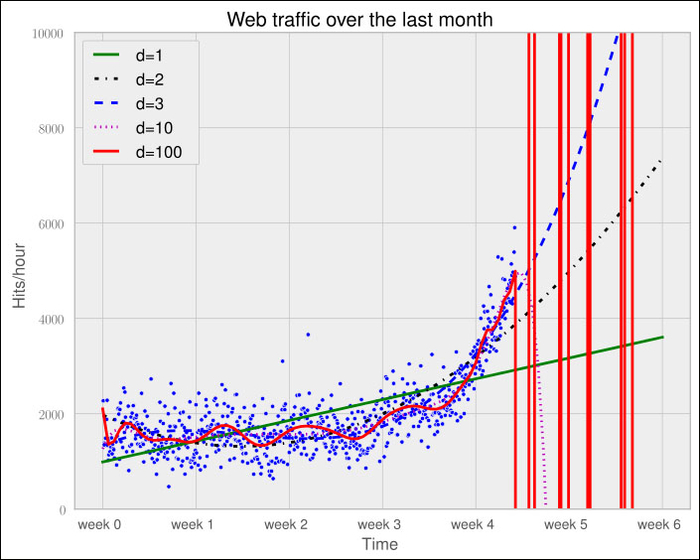The models of degree 10 and 100 don't seem to expect a bright future for our startup. They tried so hard to model the given data correctly that they are clearly useless to extrapolate further. This is called overfitting. On the other hand, the lower-degree models do not seem to be capable of capturing the data properly. This is called underfitting.

So let us play fair to the models of degree 2 and above and try out how they behave if we fit them only to the data of the last week. After all, we believe that the last week says more about the future than the data before. The result can be seen in the following psychedelic chart, which shows even more clearly how bad the problem of overfitting is: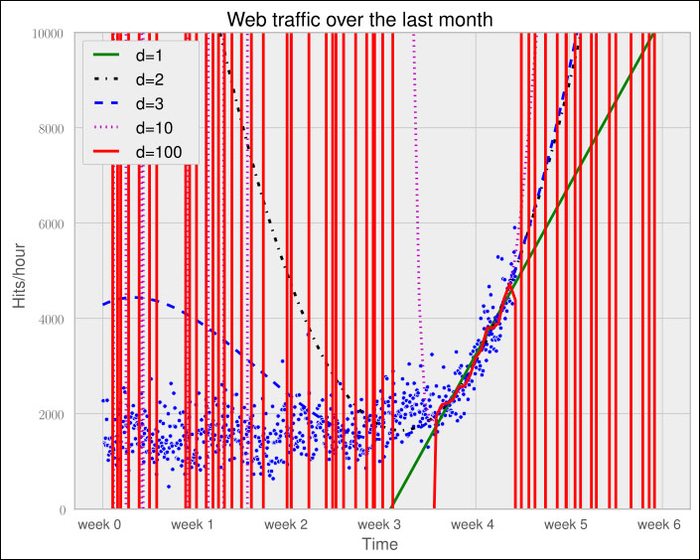Still, judging from the errors of the models when trained only on the data from week 3.5 and after, we should still choose the most complex one.

```Error d=1:   22143941.107618
Error d=2:   19768846.989176
Error d=3:   19766452.361027
Error d=10:  18949339.348539
Error d=100: 16915159.603877```

#### Training and testing

If only we had some data from the future that we could use to measure our models against, we should be able to judge our model choice only on the resulting approximation error.

Although we cannot look into the future, we can and should simulate a similar effect by holding out a part of our data. Let us remove, for instance, a certain percentage of the data and train on the remaining one. Then we use the hold-out data to calculate the error. As the model has been trained not knowing the hold-out data, we should get a more realistic picture of how the model will behave in the future.

The test errors for the models trained only on the time after the inflection point now show a completely different picture.

```Error d=1:    7,917,335.831122
Error d=2:    6,993,880.348870
Error d=3:    7,137,471.177363
Error d=10:   8,805,551.189738
Error d=100: 10,877,646.621984```

The result can be seen in the following chart: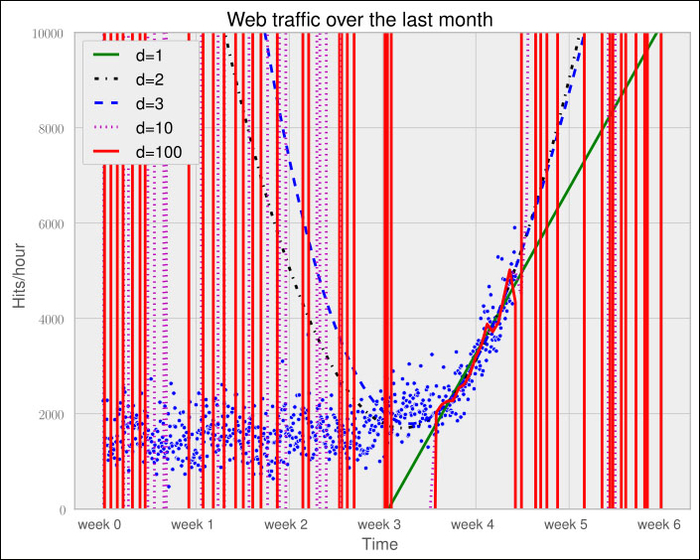It seems we finally have a clear winner. The model with degree 2 has the lowest test error, which is the error when measured using data that the model did not see during training. And this is what lets us trust that we won't get bad surprises when future data arrives.

Finally, we have arrived at a model that we think represents the underlying process best; it is now a simple task of finding out when our infrastructure will reach 100,000 requests per hour. We have to calculate when our model function reaches the value 100,000.

Having a polynomial of degree 2, we could simply compute the inverse of the function and calculate its value at 100,000. Of course, we would like to have an approach that is applicable to any model function easily.

This can be done by subtracting 100,000 from the polynomial, which results in another polynomial, and finding the root of it. SciPy's `optimize` module has the `fsolve` function to achieve this when providing an initial starting position. Let `fbt2` be the winning polynomial of degree 2:

```>>> print(fbt2)
2
0.08844 x - 97.31 x + 2.853e+04
>>> print(fbt2-100000)
2
0.08844 x - 97.31 x - 7.147e+04

>>> from scipy.optimize import fsolve
>>> reached_max = fsolve(fbt2-100000, 800)/(7*24)
>>> print("100,000 hits/hour expected at week %f" % reached_max)
100,000 hits/hour expected at week 9.827613```

Our model tells us that given the current user behavior and traction of our startup, it will take another month until we have reached our threshold capacity.

Of course, there is a certain uncertainty involved with our prediction. To get the real picture, you can draw in more sophisticated statistics to find out about the variance that we have to expect when looking farther and further into the future.

And then there are the user and underlying user behavior dynamics that we cannot model accurately. However, at this point we are fine with the current predictions. After all, we can prepare all the time-consuming actions now. If we then monitor our web traffic closely, we will see in time when we have to allocate new resources.

## Summary

Congratulations! You just learned two important things. Of these, the most important one is that as a typical machine learning operator, you will spend most of your time understanding and refining the data—exactly what we just did in our first tiny machine learning example. And we hope that the example helped you to start switching your mental focus from algorithms to data. Later, you learned how important it is to have the correct experiment setup, and that it is vital to not mix up training and testing.

Admittedly, the use of polynomial fitting is not the coolest thing in the machine learning world. We have chosen it so as not to distract you with the coolness of some shiny algorithm, which encompasses the two most important points we just summarized above.

So, let's move to the next chapter, in which we will dive deep into SciKits-learn, the marvelous machine learning toolkit, give an overview of different types of learning, and show you the beauty of feature engineering.

• ##### Willi Richert

Willi Richert has a PhD in machine learning/robotics, where he has used reinforcement learning, hidden Markov models, and Bayesian networks to let heterogeneous robots learn by imitation. Now at Microsoft, he is involved in various machine learning areas, such as deep learning, active learning, or statistical machine translation. Willi started as a child with BASIC on his Commodore 128. Later, he discovered Turbo Pascal, then Java, then C++ - only to finally arrive at his true love: Python.

Browse publications by this author
• ##### Luis Pedro Coelho

Luis Pedro Coelho is a computational biologist who analyzes DNA from microbial communities to characterize their behavior. He has also worked extensively in bioimage informatics - the application of machine learning techniques for the analysis of images of biological specimens. His main focus is on the processing and integration of large-scale datasets. He has a PhD from Carnegie Mellon University and has authored several scientific publications. In 2004, he began developing in Python and has contributed to several open source libraries. He is currently a faculty member at Fudan University in Shanghai.

Browse publications by this author

### Latest Reviews

(11 reviews total)